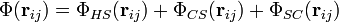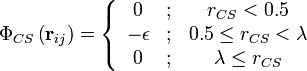# Smith and Nezbeda associated fluid modelThis article is a 'stub' page, it has no, or next to no, content. It is here at the moment to help form part of the structure of SklogWiki. If you add sufficient material to this article then please remove the {{Stub-general}} template from this page.

The Smith and Nezbeda associated fluid model  consists of a hard sphere that has an attractive square well patch. The potential is given by (Eq. 1)$\Phi({\mathbf r}_{ij}) =\Phi_{HS}({\mathbf r}_{ij}) + \Phi_{CS}({\mathbf r}_{ij}) + \Phi_{SC}({\mathbf r}_{ij})$

where (Eq. 2)$\Phi_{CS} \left({\mathbf r}_{ij} \right) = \left\{ \begin{array}{ccc} 0 & ; & r_{CS} < 0.5 \\ - \epsilon & ; & 0.5 \le r_{CS} < \lambda \\ 0 & ; & \lambda \le r_{CS} \end{array} \right.$